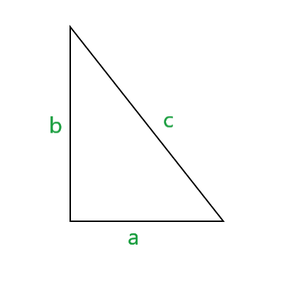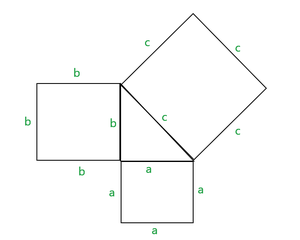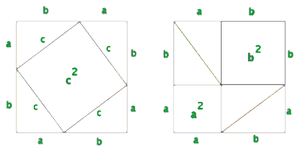Intuition behind Pythagoras Theorem

• Last Updated : 01 Jul, 2021

The Pythagoras Theorem states that in a right angled triangle, ‘a’ being the base, ‘b’ being the height and ‘c’ being the hypotenuse of  that triangle, then a2+b2=c2

Below is an illustration of this –Example –

1. if the base of a right angled triangle is 3, the height is 4,then what is the length of its hypotenuse?
Solution –  given, a=3, b=4 ,c=?
Using Pythagoras theorem,
a2+b2=c2
32+42=c2
√(9+16) =c
c=5

2. if the hypotenuse of a right angled triangle is 13, the height is 5,then what is the length of its base?
Solution –
given, a=?, b=5 ,c=13
Using Pythagoras theorem,
a2+b2=c
a2+52=132
a=√(169-25)
a=12

Intuition behind Pythagoras Theorem :
Let’s prove this theorem using the figures.
Draw squares corresponding to each side of the triangle as follows –If we look at the figure closely, we could reframe the pythagoras theorem as follows-
The area of 2 squares is equal to the third square.
ie- a2 is the area of the 1st square
b2 is the area of the 2nd square
c2 is the area of the 3rd square

thus, a2+b2=c2
Another proof of pythagoras theorem can be shown by rearranging the triangles to form 2 squares as followsIf we compare the two squares, we can find that both the squares have a+b side length, thus having the same area.
In each square, four right-angled triangles are used (realigned in a different way though)
So, we can conclude that

area(1st square) =a rea(2nd square)
c2 + 4* (area of a right angled triangle)=  a2+b2+4* (area of a right angled triangle)
c2=a2+b2  [cancelling the common terms from both sides]

Thus, pythagoras theorem is proved.

My Personal Notes arrow_drop_up